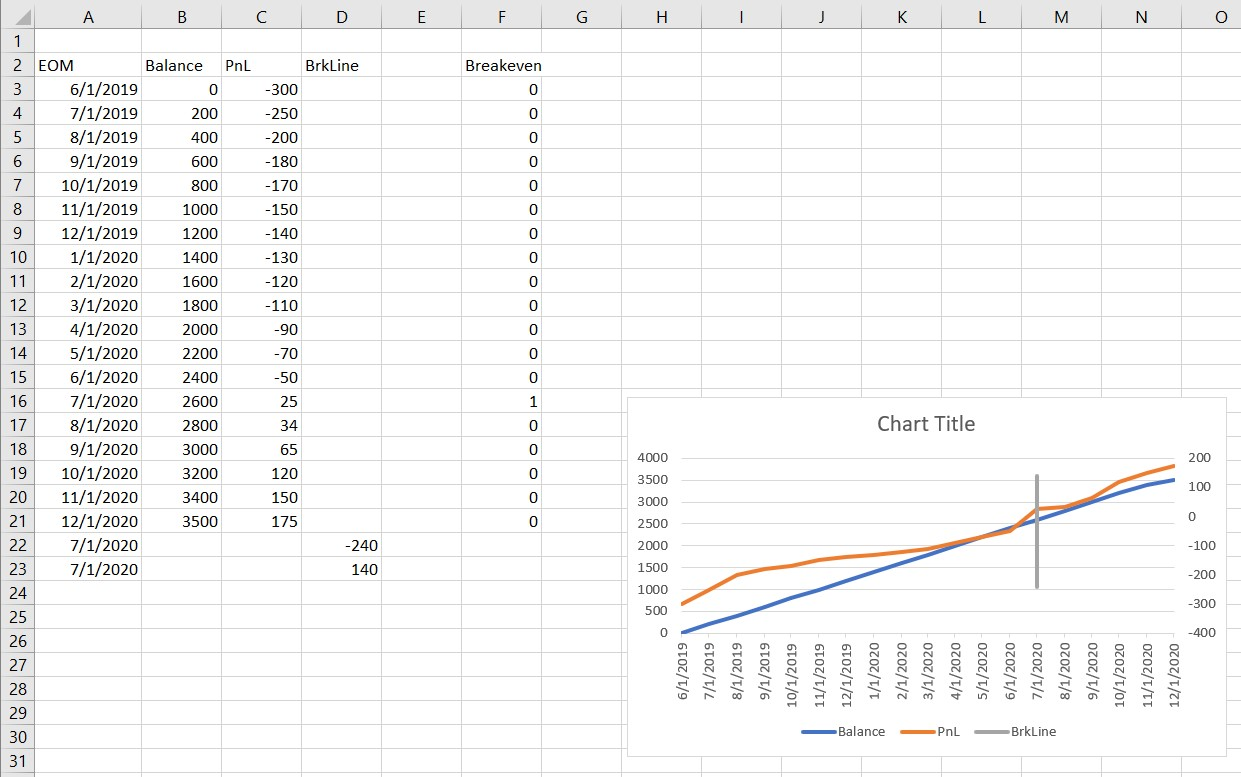# Thread: Add vertical line to chartThanks: 0Likes: 0

1. ##Add vertical line to chart

Hi,

I have a graph based on a 3-column data table where

x- axis is time (months, Jun 2019 to Dec 2020)
Primary y-axis is account balance (\$0 to \$3.5m)
Secondary y-axis is PnL (-\$300k to \$200k)

August 2020 is first month PnL > \$0 (Aug 2020 as the answer is calculated in a different cell)

How can I add a vertical line (or other indicator) to the X-axis at point August 2020 or where the line changes from -ve to +ve on the secondary axis?

TIA,
JackReply With Quote

2. ##Re: Add vertical line to chart

Here's one way which might work for you . . .

It requires you to be MANUALLY setting the Y axis minimum and maximum values, rather than leaving them to be set automatically.

Set up another chart series, and set up the data that drives that series so that BEFORE the point where you want the vertical line to appear, its value is far BELOW the Y axis minimum values, and AFTER the point where you want it to appear, its value is far ABOVE the Y axis maximum values.Reply With Quote

3. ##Re: Add vertical line to chart

You can also set this up so that the breakeven line moves based on the data. Here is an example sheet and chart.I've added another data series (BrkLine), plotted on the secondary axis, for the breakeven line. The x-axis and values for this data series are based on the data.

To know where the PnL series goes from negative to positive, I used a helper column, column F, with the cells having the formula similar to =IF(AND(C16>0,C15<0),1,0) (this is for cell F16) so there is a 1 in only the month that it turns positive.

Then cell A22 has the formula =INDEX(A3:F21,MATCH(1,F3:F21,0),1) so it is set to the same month that the PnL turned positive. Cell A23 is =A22.
For the values for the BrkLine data series, i used 80% of the minimum value of the PnL series in D22 and 80% of the maximum for the PnL series in D23. This keeps the line within the vertical space of the secondary axis and adjusts if the data changes.

This way, the breakeven line moves and gets longer or shorter as the data changes.

One last thing I did is change the setting in the Hidden and Empty Cells dialog box in the Select Data dialog box to have Excel connect data points if there is am empty cell. This keeps the Balance and PnL lines unbroken.

Hope this helps.Reply With Quote

4. ##Re: Add vertical line to chart

Thanks both @Gerald Higgins and @Dave Paradi, Dave I will adapt your approach as I believe it'll fit my setup up easier and I'm already using an almost equivalent formula to find the month breakeven occurs in.

FYI for the helper column, try this formula instead:
Code:
`=--(AND(C15<0,C16>=0)`
"--" converts TRUE into 1 and FALSE into 0, yes my mistake I did mean including breakeven, i.e. 0 or higher, NOT when profit is only positive (i .e. > 0)

Cheers,
JackReply With Quote

## User Tag List

#### Tags for this Thread

august, axis, line, pnl, secondary####Posting Permissions

• You may not post new threads
• You may not post replies
• You may not post attachments
• You may not edit your posts
•